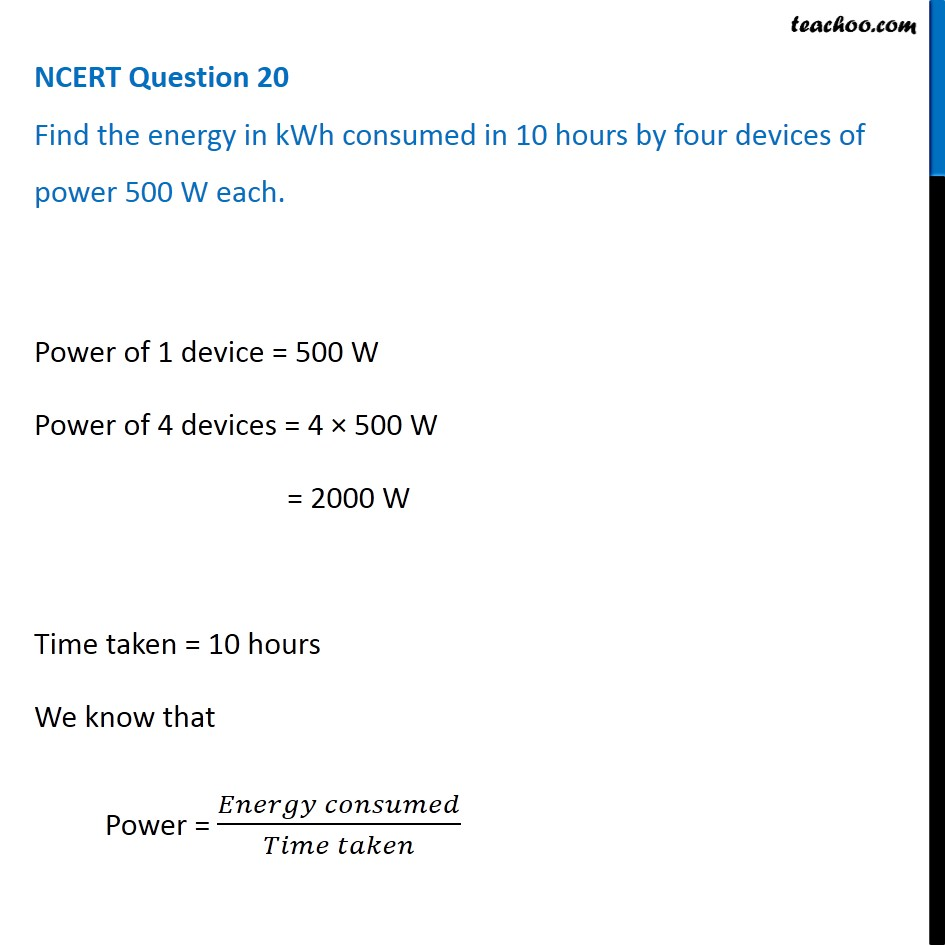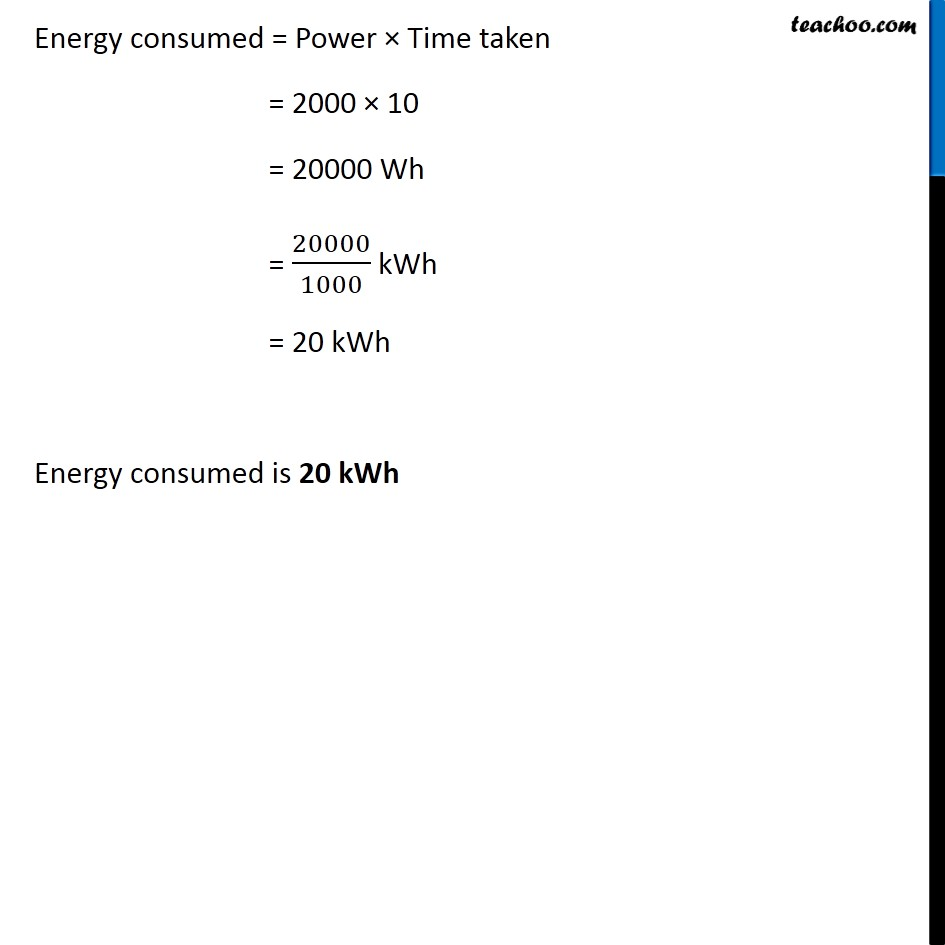NCERT Questions

Class 9
Chapter 11 Class 9 - Work and EnergyLearn in your speed, with individual attention - Teachoo Maths 1-on-1 Class

### Transcript

NCERT Question 20 Find the energy in kWh consumed in 10 hours by four devices of power 500 W each. Power of 1 device = 500 W Power of 4 devices = 4 × 500 W = 2000 W Time taken = 10 hours We know that Power = (𝐸𝑛𝑒𝑟𝑔𝑦 𝑐𝑜𝑛𝑠𝑢𝑚𝑒𝑑)/(𝑇𝑖𝑚𝑒 𝑡𝑎𝑘𝑒𝑛) Energy consumed = Power × Time taken = 2000 × 10 = 20000 Wh = 20000/1000 kWh = 20 kWh Energy consumed is 20 kWh